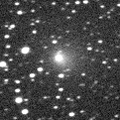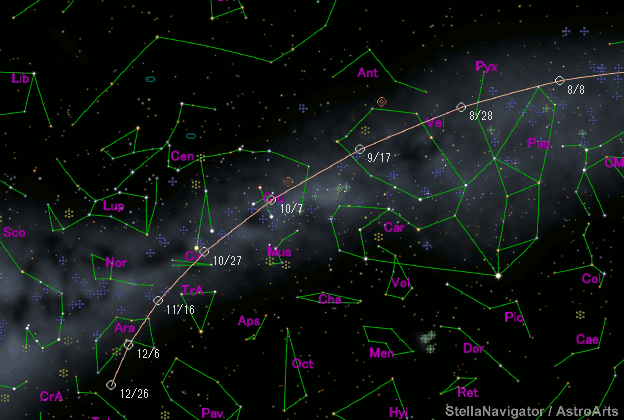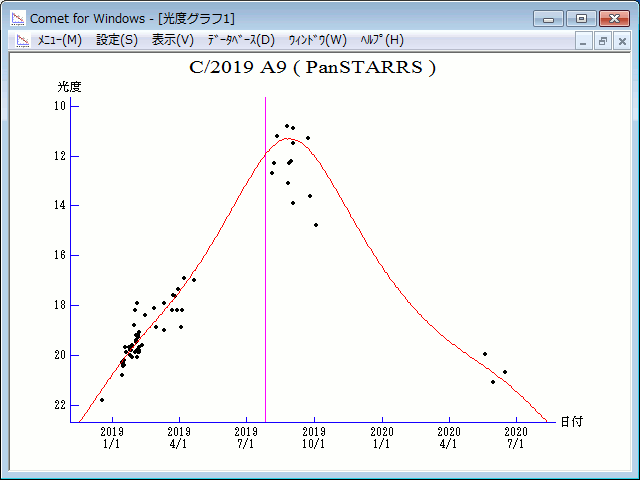# \$B%Q%s%9%?!<%:WB@1(B

C/2019 A9 ( PanSTARRS )###\$B%W%m%U%#!<%k(B

 \$BH/8+F|(B 2019\$BG/(B1\$B7n(B14\$BF|(B \$BH/8+8wEY(B 20.3\$BEy(B \$BH/8+ Pan-STARRS 1 telescope (Haleakala)

###\$B###\$B50F;MWAG(B

```Epoch 2019 July 16.0 TT = JDT 2458680.5
T 2019 July 26.52568 TT                                 Rudenko
q   1.4263969            (2000.0)            P               Q
z  +0.0257937      Peri.  237.80144     -0.16009678     +0.07117690
+/-0.0000000      Node   278.36848     +0.80750013     -0.56420356
e   0.9632080      Incl.   84.34157     -0.56772578     -0.82256197
From 185 observations 2018 Dec. 18-2020 June 16, mean residual 0".4.
```

###\$B@1?^(B###\$B8wEYJQ2=(B

```        m1 = 6.0 + 5 log\$B&\$(B + 25.0 log r(t - 30)  [  ,30]  (              \$B!A(B2019\$BG/(B 8\$B7n(B25\$BF|(B)
m1 = 6.8 + 5 log\$B&\$(B + 20.0 log r(t - 30)  [30,  ]  (2019\$BG/(B 8\$B7n(B25\$BF|!A(B              )
```##### \$B50F;MWAG\$O(BM.P.E.C. 2020-P19\$B\$K7G:\\$5\$l\$?\$b\$N\$G\$9!#(B \$B@1?^\$O%9%F%i%J%S%2!<%?(B Ver.10 (\$B%"%9%H%m%"!<%D(B) \$B\$G:n@.\$7\$?\$b\$N\$G\$9!#(B \$B8wEY%0%i%U\$O(BComet for Windows\$B\$G:n@.\$7\$?\$b\$N\$G\$9!#(B